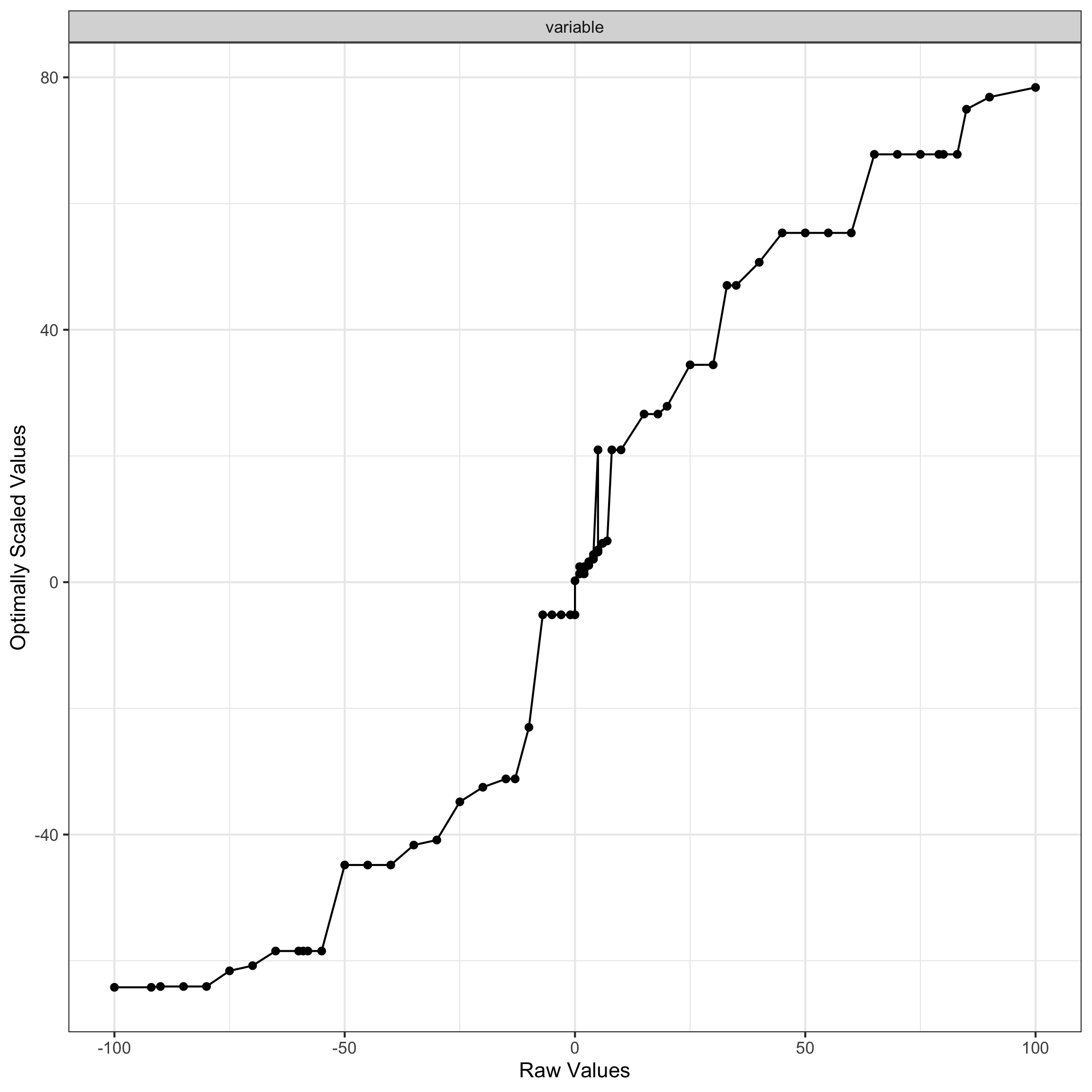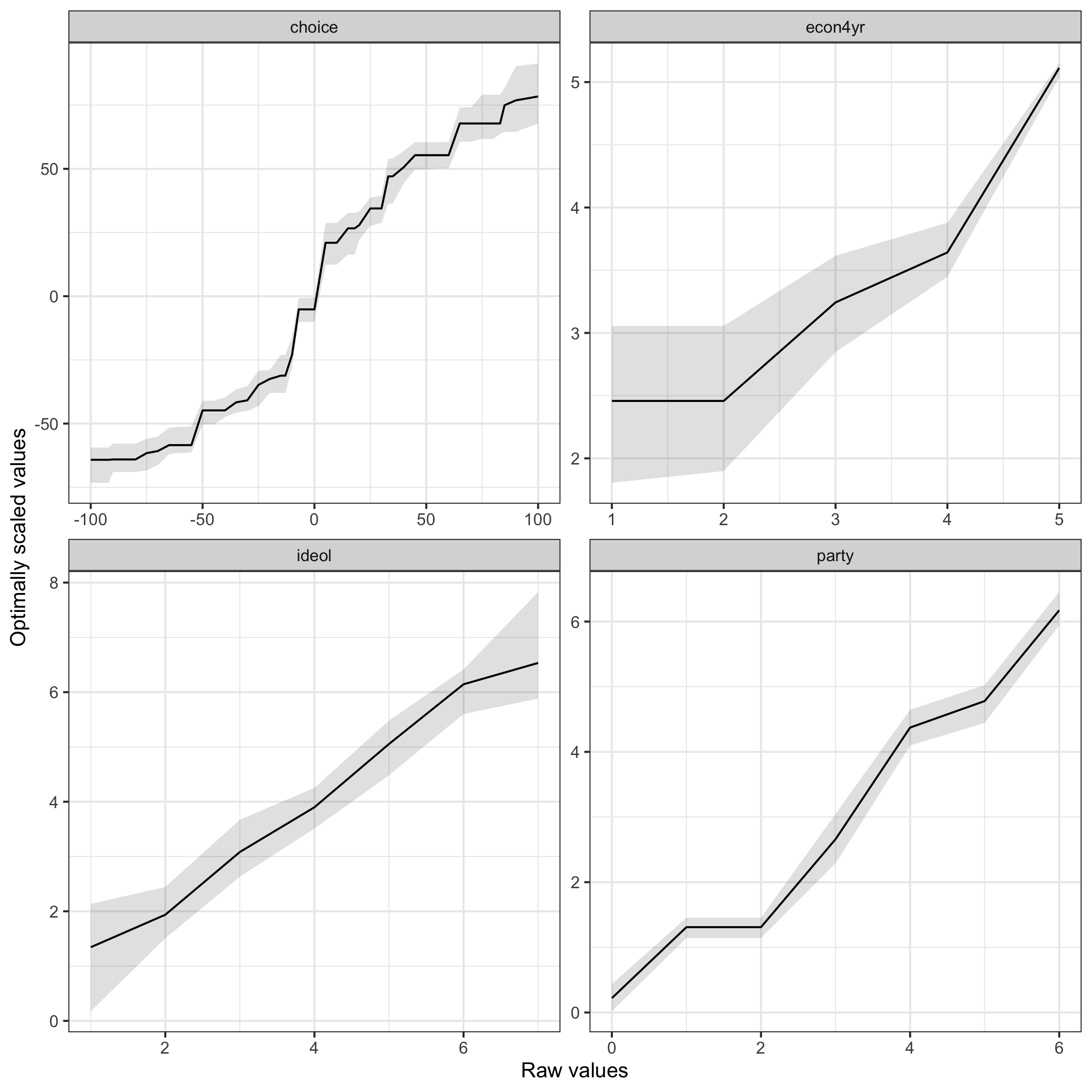Estimating ALSOS Models

2022-01-11

As of version 1.6, the {DAMisc} package has a more complete version of the ALSOS model. This model allows qualitative variables to be optimally scaled to maximize fit in a linear model. The package also includes a function to bootstrap the ALSOS model to permit visualization of uncertainty around the transformed variable values. Below, we walk through how to use and interpret the functions.

To demonstrate the function, we use the elec92 data from the {optiscale} package:

data(elec92, package="optiscale")

We are estimating a model of choice (a difference in respondent feeling thermometer scores for Bush and Clinton), party (a seven-point party-identification measure), econ4yr retrospective national economic evaluations (five-point scale) and ideol (self-placement on seven-point liberal-conservative scale). For more information, see the help file for the data in the {optiscale} package.

The ALSOS model can be estimated with the alsos() function in the {DAMisc} package:

library(DAMisc)
mod <- alsos(choice ~ party + ideol + econ4yr, data=elec92, scale_dv=TRUE)

The best way to interpret the results is to visualize them with the plot() function:

plot(mod)The function to bootstrap the ALSOS model uses a non-parametric bootstrap (i.e., bootstrapping the observations) and estimates the model once for each bootstrap sample. The unique transformed values are saved and returned either in a bootstrap object or in a data frame with their original values and percentile confidence intervals (or both). One important feature of this bootstrap is that the bootstrap samples are stratified on the dependent variable to ensure that the dependent variable will maintain the same mean and variance across the bootstrap samples. Ideally, this would be done across all combinations of the variables being scaled to keep their properties constant across the bootstrap samples, but when there are lots of values (as with the choice variable here) half of the bootstrap observations would be singletons and thus they would show up in all bootstrap samples thus underestimating variability for that particular group.

In the output below, we use just 50 bootstrap samples in the interest of speed, but in “real life” you would likely want to use many more than that (perhaps around 2500).

library(boot)
boot.mod <- boot.alsos(choice ~ party + ideol + econ4yr, data=elec92, level=2, R=50)
library(ggplot2)
ggplot(boot.mod\$data, aes(x=raw_vals, y=os_vals)) +
geom_ribbon(aes(ymin=lower, ymax=upper), alpha=.15) +
geom_line() +
facet_wrap(~variable, scales="free") +
theme_bw() +
labs(x="Raw values", y="Optimally scaled values")